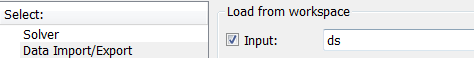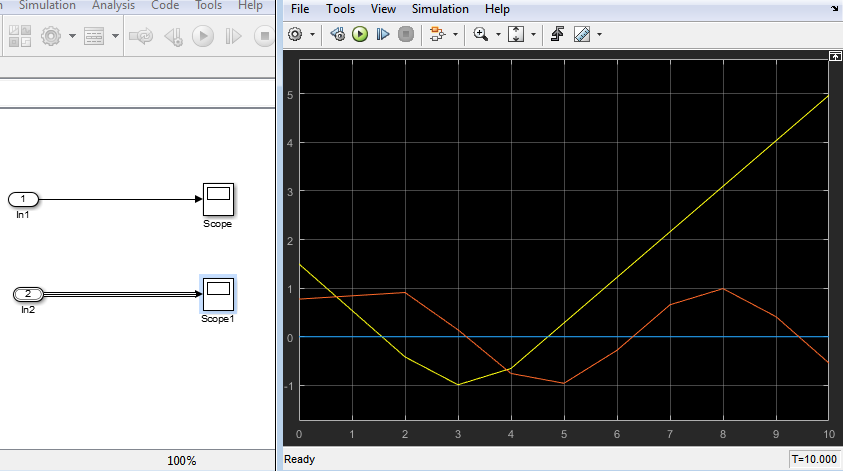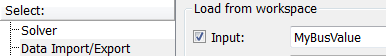Documentation

Load Bus Data to Root-Level Input Ports

You can import bus data to top-level input ports by manually specifying the data in the Input configuration parameter or by using the Root Inport Mapper tool. For information about importing bus data using the Root Inport Mapper tool, see Import Bus Data.

Imported Bus Data Requirements

You can import bus (virtual, nonvirtual, or array of buses) data to a top-level input port defined by a bus object (see Simulink.Bus). In the top-level Inport block, set Data type to Bus and specify the name of a bus object. To specify data values for bus signals, use a structure of:

• MATLAB® timeseries objects

• MATLAB timetable objects

• A combination of timeseries and timetable objects

Bus elements for which you do not include a field in the structure use ground values. You can use an empty matrix to specify to use ground values.

Note

When you specify timetable data to load, the timetable must contain data for only one signal.

The structure of timeseries or timetable (or both) objects must match the bus elements in terms of:

• Hierarchy

• Name of the structure field, which must match the bus element name. (The name property of the timeseries object does not need to match the bus element name.)

• Data type

• Dimensions

• Complexity

The order of the structure fields does not have to match the order of the bus elements.

You can include the structure as an element of a Dataset object. You can use a structure in a comma-separated list. You can specify an empty matrix in a comma-separated list. The empty matrix uses the ground values for the bus signal.

For example, to load data for input ports in1 and in3, and to use ground values for port in2, enter the following in the Input parameter:

in1, [], in3

Note

If you use a structure of MATLAB timeseries objects for a root Inport block in a model with multiple root Inport blocks, all root Inport blocks must use MATLAB timeseries or timetable objects. Convert any root Inport block data that uses Simulink.TsArray or Simulink.Timeseries objects to MATLAB timeseries objects.

Initialize Bus Signals

You can initialize bus signals, including using partial specification of initialization data. For details, see Specify Initial Conditions for Bus Signals.

For details about importing array of bus data to a root Inport block, see Import Array of Buses Data.

Limitations for Importing Bus Data to Top-Level Inputs

The signal data that you can use the Root Inport Mapper tool to import and map to a top-level Inport block can include bus data. You cannot use that tool to map bus signals to a top-level Enable or Trigger block.

You cannot use input ports to import buses in external modes. To import bus data in rapid accelerator mode, use Dataset format.

Import Bus Data to a Top-Level Inport

This model has two Inport blocks connected to Scope blocks. The data type of the In1 block is inherited (nonbus data) and the data type of the In2 block is defined by the bus object BusObject. The model has a callback that loads BusObject and its sub-bus BusObject1.The BusObject bus object has two elements:

• c

• s1, which is a nested bus that has two elements:

• a

• b

1. Open the model.

2. Create a MATLAB timeseries object for In1, for which you want to import nonbus data.

For example:

t1 = (1:10)';
d1 = sin(t1);
in1 = timeseries(d1,t1);
3. Create an input structure, which can consist of MATLAB timeseries objects or MATLAB timetable objects, or a combination of those types of objects. Create one timeseries or timetable object for each leaf bus element for which you do not want to use ground values. This example uses ground values for the b bus element, so it does not need a timeseries or timetable object for that element.

t2 = (1:5)';
d2 = cos(t2);
in2.c = timeseries(d1,t1);
in2.s1.a = timetable(seconds(t2),d2);

The MATLAB timeseries objects that you create must match the corresponding bus elements, as described in Imported Bus Data Requirements.

4. Create a Dataset object and add in1 and in2 to the data set.

5. In the Configuration Parameters > Data Import/Export > Input parameter edit box, enter the Dataset object ds.6. Simulate the model. The Scope block connected to In2 shows the imported bus data.Get Information About Bus Objects

To determine the number of MATLAB timeseries objects and data type, complexity, and dimensions needed for creating a structure of timeseries objects from a bus, use these methods:

For example, for the bus object BusObject:

num_el = BusObject.getNumLeafBusElements
num_el =

3
el_list = BusObject.getLeafBusElements
el_list =

3x1 BusElement array with properties:

Min
Max
DimensionsMode
SampleTime
Description
Units
Name
DataType
Complexity
Dimensions
el_list(1).Dimensions
ans =

1

Create Structures of Timeseries Objects from Buses

If you have timeseries objects defined, you can use them to create a structure of timeseries objects based on a bus object. Use the Simulink.SimulationData.createStructOfTimeseries function. For example, if you have defined timeseries objects ts1, ts2, and ts3, and you have a bus object MyBusObject, you can use this command to create a structure of timeseries objects:

'MyBusObject',{ts1,ts2,ts3});

The number of timeseries objects in the cell array must match the number of leaf elements in the bus object. The data type, dimensions, and complexity of each timeseries object must match those attributes of the corresponding bus object leaf node.

Import Array of Buses Data

To import (load) array of buses data using a root Inport block, use an array of structures of MATLAB timeseries objects.

Note

You cannot use an Enable, Trigger, From Workspace, or From File block to import data for an array of buses.

Full Specification of Data

You can use logged data for an array of buses signal from a previous simulation as roundtrip input to a root-level Inport in a subsequent simulation run. The logged data is a full specification of data for the Inport block.

If you construct an array of structures of MATLAB timeseries objects to specify fully the data to import:

• Specify the structure fields in the same order as the signals in the bus signals.

• Do not include more fields in the structure than there are signals in the bus.

For leaf fields, match exactly the data type, dimensions, and complexity of the corresponding signal in the bus.

Partial Specification of Data

To specify partial data for array of buses, create a MATLAB array of structures with MATLAB timeseries objects at the leaf nodes.

The structure that you create to specify partial data must be consistent with these rules:

• You can omit fields, including leaf nodes and subbranches. You can also omit dimensions. If you do not specify a field, Simulink® uses the ground value for that field.

• For nested bus nodes, make the dimension of each field equal to, or smaller than, the dimension for the corresponding node of the array of buses.

This example shows how you can specify partial data to be imported using a root Inport block whose data type is defined as bus object MyBus. You can open the model (ex_partial_loading_aob_model) and the MATLAB code that defines the data to import (ex_partial_loading_aob_data.m).The input Inport block uses the MyBus bus object as its data type.The MyBus array of buses includes MyBus(1) and MyBus(2). The port dimension is set to 2 to reflect the two buses in the array of buses, and Output as nonvirtual bus is enabled.

Here are the elements of the array of buses, which includes MyBus(1) and MyBus(2). The color highlighting shows the nodes of the array of buses for which data is being imported.Here is MATLAB code that defines the data to import. The color that highlights the code matches the color of the corresponding node in the array of buses. To view the code used in this model, open the MATLAB code file ex_partial_loading_aob_data.m.In the code that defines the import data:

• The timeseries object MyBusValue specifies the data for the highlighted nodes.

• The timeseries object BT for MyBus(2), because BT is a leaf node, it must match exactly the dimensions, data type, and complexity of the corresponding bus element.

• The structure specifies data for Y(2). You can skip the first and last nested buses of Y (that is, Y(1) and Y(3)).

This example specifies data for Y(2); you can skip the first and last nested buses of Y (that is, Y(1) and Y(3)).

After you define the MyBusValue variable for the import data, set the Configuration Parameters > Data Import/Export > Input parameter to MyBusValue.